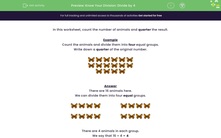# Know Your Division: Divide by 4

In this worksheet, students count the number of animals and divide them into quarters (in other words, divide by 4).Key stage:  KS 2

Curriculum topic:   Number: Multiplication and Division

Curriculum subtopic:   Use Multiplication/Division Facts (3, 4 and 8)

Difficulty level:#### Worksheet Overview

In this worksheet, count the number of animals and quarter the result.

Example

Count the animals and divide them into four equal groups.

Write down a quarter of the original number.There are 16 animals here.

We can divide them into four equal groups.There are 4 animals in each group.

We say that 16 ÷ 4 = 4

### What is EdPlace?

We're your National Curriculum aligned online education content provider helping each child succeed in English, maths and science from year 1 to GCSE. With an EdPlace account you’ll be able to track and measure progress, helping each child achieve their best. We build confidence and attainment by personalising each child’s learning at a level that suits them.

Get started